# Data Structures for Beginners | A Complete Guide

Written By Preethi. M

## Introduction:

Most of us see a question and just start typing out some logic that comes to our mind. Most of the time we don’t give importance to which approach is to be followed. While a program can have many possible answers, there is only a few which is optimal. As good programmers our aim is to find the most optimal solution.

So how can we do this?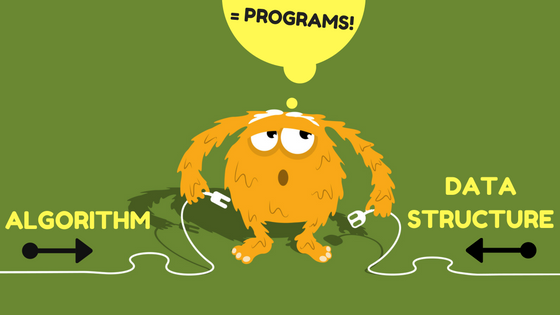That's right!! The answer is `Data Structures and Algorithms`!!!.
With these two ‘weapons’ we can solve any complex question and also make our approach optimal.

In this post we are going to cover the basics about Data Structures.

## Topics covered:

1. Importance of Data Structures
2. Sample statistic to show need for Data Structures
3. List of Data Structures

Each Data structure is a vast topic by itself, due to this reason many topics are only discussed on a very basic level and there is a lot more to learn about them.

So grab your cup of coffee and dive in!!!

Let's start off with the main question.
Why should I use Data Structure in my logic?

Imagine you have a whole rack of books scattered everywhere without any specific order or arrangement. You would take a really long time to search for one book right.
But what if you took a little extra time to arrange it in the right position? Wouldn’t it save you a lot of time in all future searches for a book.

That’s what data structures are about. It makes the data more organised for the purpose and makes future operations on it easier and faster, provided the data is inserted in the right order (i.e., using the appropriate data structure)
It is very important to choose the right structure based on the need and application. We should consider which operation is done the most and choose a structure which can optimise that operation.

## Big O Notation

To compare the efficiency of different data structures we use something known as the Big O Notation.

Big O Notation is widely used to measure the efficiency of an algorithm in terms of its time and space requirements.
`Time complexity` is the computation time taken for the code to execute as the input size increases.
`Space complexity` is the amount of memory used for its execution.

• Linear complexity O(n): means that for an input of size 'n' we have the code performing 'n' instructions.
• Quadratic complexity O(n2): means that for an input of size 'n' the code performs 'n2' instructions.
• Constant complexity O(1): means that for an input of size 'n' the code performs only a constant number of instructions irrespective of the size of input. This gets very efficient when the size is very large.

All the complexity tables discussed further are the average case complexities as worst case are the upper bound and efficiency of a structure cannot be appreciated and compared using worst case.

Now that we are clear with complexities, let's go ahead with some statistics.

## Let's take a simple example:

->You want to search for some of your favourite books in your large collection of books(example of insert once, search multiple times)

### Approach 1: Using List structure

You had arranged all yours books in a random order in your racks. Now you will have to search the collection linearly to find the book and worst still if you had forgotten that your friend had borrowed that book, you will end up searching the entire collection for no reason.
`Time complexity:O(n)`[linear complexity]

### Approach 2: Using Binary Search Tree (or binary search on sorted list

Say you had arranged the books in alphabetical order. You can check the middle book first, if it comes after the name of your favourite book then search the first half, or else search the second half(known as `decrease and conquer` method).
You have now reduced the time complexity to `O(log n)` [logarithmic time complexity]

Now if you are familiar with time complexities you may question how is this efficient if it is not sorted cause major sorting techniques take a time of O(n log2n) and then an additional O(log2n) for binary search while searching linearly would take only O(n)

Here is where the matter of which operation is performed the most comes into play, in this example sorting would be done once but we are doing search operation multiple times, in the long run Binary search proves to be more efficient than linear search.

### Approach 3:Using Hashmap or Hashset (the most efficient for unique data)

Now hashmaps is a data structure that can be used for unique data or data with unique keys.
If your library racks had a number for each position and you were able to find a unique value(called hash value) for each book using some function(called hash function) then you can place it in that position. Now when you want to search for your book all you have to do is find the hash value from the hash function and go to that position and take your book.
We have now found a way to search the book in constant time independant of your total number of books.
This is called constant time complexity, denoted by `O(1)`.

Hashset is similar to hashmap except the fact that hashmap always points to a unique key(has key-value pairs) but hashset can have same hashcode(stored as object, no key-value pairs) for two elements leading to collisions.This is why hashmaps are faster than hashsets.

Time taken for 106 search queries in a dataset of 104 unique elements

Now that we have understood the need for Data Structures let's dive into the various types of data structures!

### Data Structures are mainly of two types:

->Linear(Eg: list,stack,queue,hashmap(dictionary in python),hashset(sets in python))

In this type, the elements may be placed randomly or sequentially in the computer memory based on the implementation, but the access is always sequential.

->Non-Linear(Eg: Tree,Graph)

In this type, the elements may be placed randomly or sequentially in the computer memory and even the access can be sequential or random.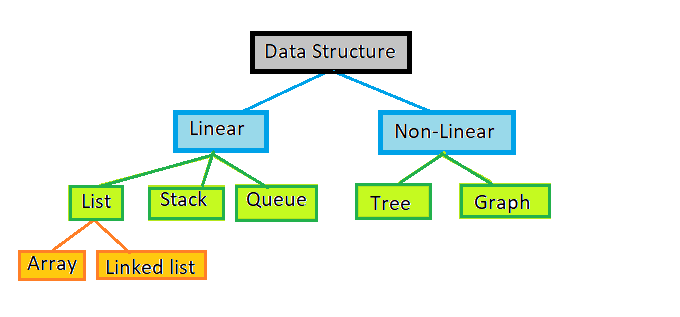For all the data structures the main operations that we can perform are: `create`,`insert`,`delete`,`search`,`destroy`.

### 1.List

A basic structure where all the elements are always accessed sequentially.
Uses:
This structure can be used when we want to access all the elements in a sequential manner with lesser memory consumption.
It is mostly used to implement other data structures and it offers very limited use by itself.

A list can be implemented by two ways:

1. Array: the storage is sequential in the memory.
2. Linked list: the storage in memory can be random, this can be achieved by linking the current to next with a pointer(this leads to additional memory requirement).

Linked list are of various types like singly linked list, doubly linked list, circular singly linked list, etc, which are not going to be discussed in detail in this post.

Time complexity of every structure depends on the implementation of the structure.

#### Time complexity of lists:

SearchO(n)O(n)
InsertO(n)O(1)
DeleteO(n)O(1)
AccessO(1)O(n)

Space complexity: `O(n)`

Space required to implement list using linked list is more precisely O(2n) due to additional pointers but it is approximated to be O(n).

### 2.Stack

A list with a top pointer makes a stack. This list can be accessed or modified only through the top pointer and the other elements remain inaccessible.
This structure is used when we want to do a last in first out operation (LIFO).
Push means inserting into a stack and pop means removing it from the stack. Peek means just viewing the top of the stack.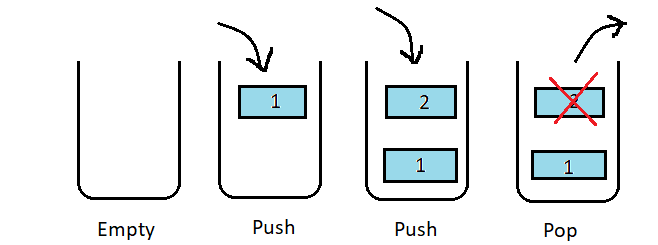Uses:

1. Recursive stack maintained by the program between calls.
2. Infix to prefix or postfix conversion.
3. Undo and redo in editors.
Time and space complexity depends on the implementation of stack(using array or linked list).

### 3.Queue

A list with a front and rear pointer makes a queue structure. This structure can only be modified via its front or rear pointers while the others remain inaccessible.

Various implementations of queues are possible like simple queue, circular queue, double ended queue, priority queue, etc. (The only difference is how the front and rear pointers are defined and what they perform).

Enqueue means pushing into the queue from the rear end and dequeue means taking an element out from the front end.
A queue is a first in first out(FIFO) structure.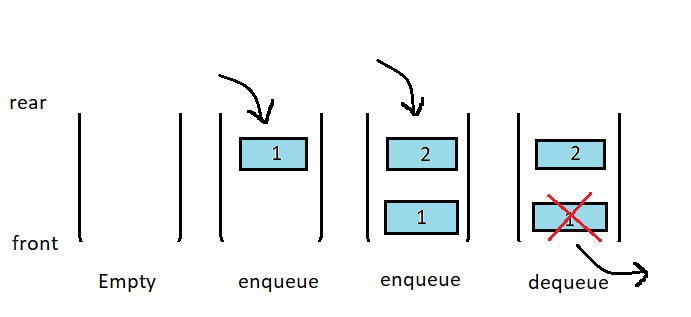Uses:

1. CPU scheduling
2. Binary tree traversal(iterative version)

Just like stacks the queue structure can also be implemented using lists and have their corresponding complexities.

### Tree

This is one of the most important structure and has wide range of uses, even the files stored in your system uses a tree structure.
Trees have various sub-structures which can be used as per the need.
E.g.: AVL, splay tree, RBT (red black tree), BST (binary search tree), Trie tree, heaps, B Trees.
Due to the vastness of the topic, these won’t be discussed in detail.

Uses:

• AVL:
A balanced tree which can be used for cases with few insertions and large search queries.

O(log2n)-for search

• Splay Tree:
A structure which can be used to move the most recent search to the top thus making it easier to search it in the future.

E.g.:In hospital records: a record which is searched once will be needed more frequently than those of discharged patients so moving this to a more easier to search position reduces the time for search queries.

• BST:
A binary tree in which the root's value is greater than each left subtree and lesser than each right subtree makes a binary search tree.Inserting is time comsuming but search complexity is logarithmic so it is used for scenarios with few insertions and multiple searches.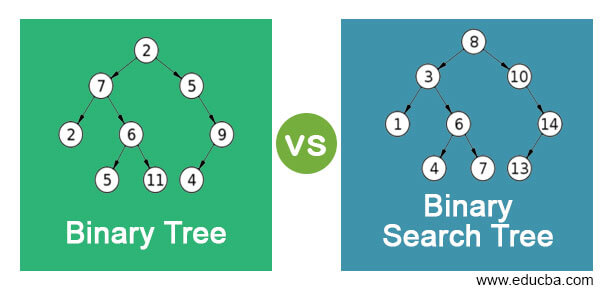#### Time complexity of BST:

OperationAverage Time Complexity
SearchO(log2n)
InsertO(log2n)
DeleteO(log2n)
AccessO(log2n)

Space complexity: `O(n)`

• Trie Tree:
Trie tree (also known as prefix tree or digital tree) is a type of k-ary search tree which can be used for searching keys.
Using BST to search for a word of length M in a set of N keys then time time complexity would be `O(M*log N)`[for a well balanced BST], but using Trie we can bring it down to `O(M)`.
But at what cost do we get this benefit?
The space complexity of a Trie.

While most structures take linear or logarithmic space, trie trees have quadratic space complexity because of all the pointers it holds for each key.

Trie can be used for autocorrect features, dictionaries and substring matching.• Heaps:
A tree should have two properties to be called a heap.
1. Structural property

the tree should be a complete binary tree.

1. Value property(parental dominance)

all the parent nodes should have a value greater than the child nodes(if maxheap) or all the parent nodes should have value lesser than the child nodes(if minheap).

It is a tree structure which can be used for sorting (heap sort) and priority implementation (priority queues).

• B Trees: It is a balanced tree which makes the execution speed to retrieve information from the disc faster by increasing the number of children.

A balanced binary tree reduces the time complexity of search to O(log2n), similarly a balanced tree with 'm' child nodes can do the search in O(logmn). This forms the basis of B Trees.### Hashmap

A hashmap is created by calculating a hash value for each element and using it to place it in the hashmap. When searching for an element, the hash value can be calculated and searched in constant time.
This structure can be used to get the fastest search result cause it can search in constant time (lots of collisions can make it O(n) which is very unlikely).

Collision occurs when two or more elements have the same hash value. Collision handling is done by linear probing, quadratic probing, double hashing, separate chaining or rehashing.
Rehashing is done when the space in the map is not enough. To maintain an optimal hashmap the load factor(load factor = number of elements/total size of hashmap) is set to 0.75 which ensures minimum collisions.

#### Time complexity of Hash Table:

OperationAverage Time Complexity
SearchO(1)
InsertO(1)
DeleteO(1)
AccessN/A

Space complexity: `O(n)`

### Graph

A graph is a set of nodes(vertices) connected by edges. A graph can be cyclic or acyclic, directed or undirected, weighted or unweighted, connected or not connected.

A graph can be implemented using any of the following:

A graph can be traversed by:
`Depth First Search(DFS)`

Check connectivity of graph, check if path exists between two vertices, check number of components in the graph.

`Breadth First Search(BFS)`

Helps to get the shortest path between two vertices and can do everything that a DFS traversal can do.

Uses:
Graphs can be used in computer networks, social analysis and shortest path problems.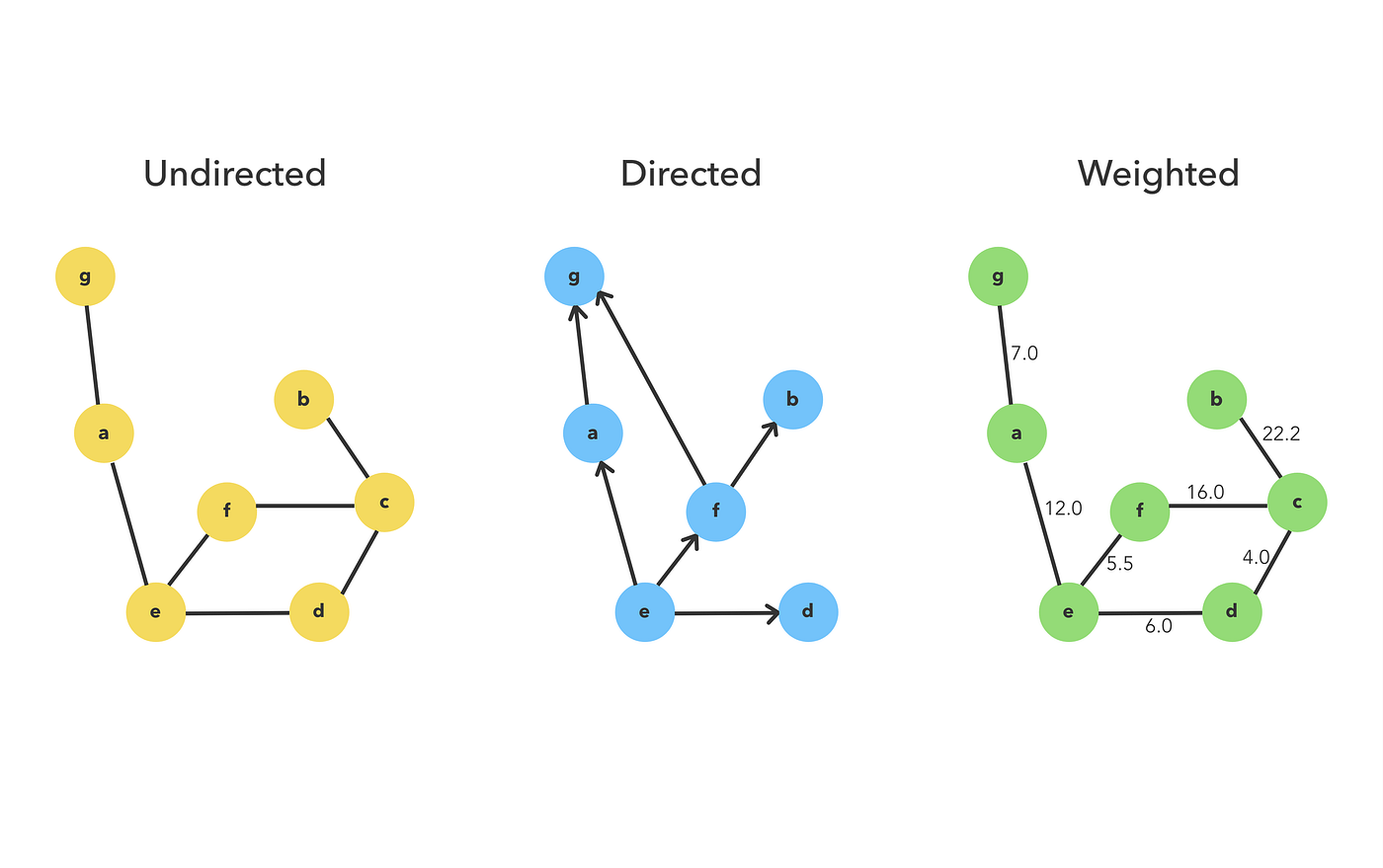Data structures by itself is a very extensive topic and I have tried my best to keep it short and to the point but data structures have a lot more to offer and everything mentioned above is just a bird's view of the vast world of data structures.

Kudos to those who had the patience to read the entire post.

I would like to conclude the blog with a famous quote by Linus Torvalds.

`"Bad programmers worry about the code. Good programmers worry about data structures and their relationships."`